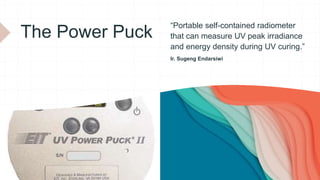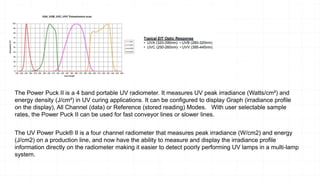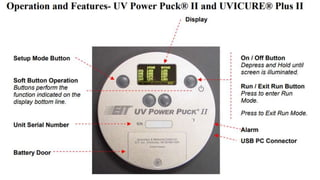Diese Präsentation wurde erfolgreich gemeldet.

# UV Expose Measurement

Anzeige
Anzeige
Anzeige
Anzeige
Anzeige
Anzeige
Anzeige
Anzeige
Anzeige
Anzeige
Anzeige×

1 von 8 Anzeige

# UV Expose Measurement

This is a value for energy dose and accurately describeas the total
accumulative exposure of UV received. It is W/cm2 multiplied by time.

This is a value for energy dose and accurately describeas the total
accumulative exposure of UV received. It is W/cm2 multiplied by time.

Anzeige
Anzeige

### UV Expose Measurement

1. 1. The Power Puck “Portable self-contained radiometer that can measure UV peak irradiance and energy density during UV curing.” Ir. Sugeng Endarsiwi
2. 2. The Power Puck II is a 4 band portable UV radiometer. It measures UV peak irradiance (Watts/cm²) and energy density (J/cm²) in UV curing applications. It can be configured to display Graph (irradiance profile on the display), All Channel (data) or Reference (stored reading) Modes. With user selectable sample rates, the Power Puck II can be used for fast conveyor lines or slower lines. The UV Power Puck® II is a four channel radiometer that measures peak irradiance (W/cm2) and energy (J/cm2) on a production line, and now have the ability to measure and display the irradiance profile information directly on the radiometer making it easier to detect poorly performing UV lamps in a multi-lamp system.
3. 3. Let’s start with a short glossary – following is a list of the most common terms typically used to describe a UV Bulb’s power or intensity. (Source: https://www.uvprocess.com/) Watts This is the total amount of energy the bulb will use at 100% power. Unfortunately, it is not a useful indication for UV because UV lamps are extremely inefficient. Total watts include: 1. White Light 2. Infra Red (IR) Heat 3. substantially low proportion of Ultra-Violet (UV) energy. (~92% waste energy and ~8% UV) WPI “Watts per Inch”; this is the same as Watts but it is broken down into each linear inch of the UV Arc Lamp. An example would be as follows: You have a 10 inch arc lamp that operates at 300WPI. 10 inches of arc X 300 per inch = 3,000 Watts. (In some parts of the world, W/cm = Watts per centimeter)
4. 4. W/cm2 This is a value for energy intensity and if the correct UV Radiometer was used; it will accurately describe the peak output power of a UV Lamp. Intensity can be also expressed in lower levels as mW/cm2 (milliwatts) or even lower as µW/cm 2 (micro-watts). The portion at the end (/cm2) stands for per centimeter squared, or per square centimeter. It represents the detector size used within the radiometer. All radiometers calculate based on a square centimeter detector so that there is some uniformity within the industry. J/cm2 This is a value for energy dose and accurately describes the total accumulative exposure of UV received. It is W/cm2 multiplied by time. Similar to W/cm2, in lower doses it can be represented as mJ/cm2 (millijoules) or even lower as µJ/cm2(micro-joules). Note: 1 Joule = 1 W/cm2 /second. (Source: https://www.uvprocess.com/)